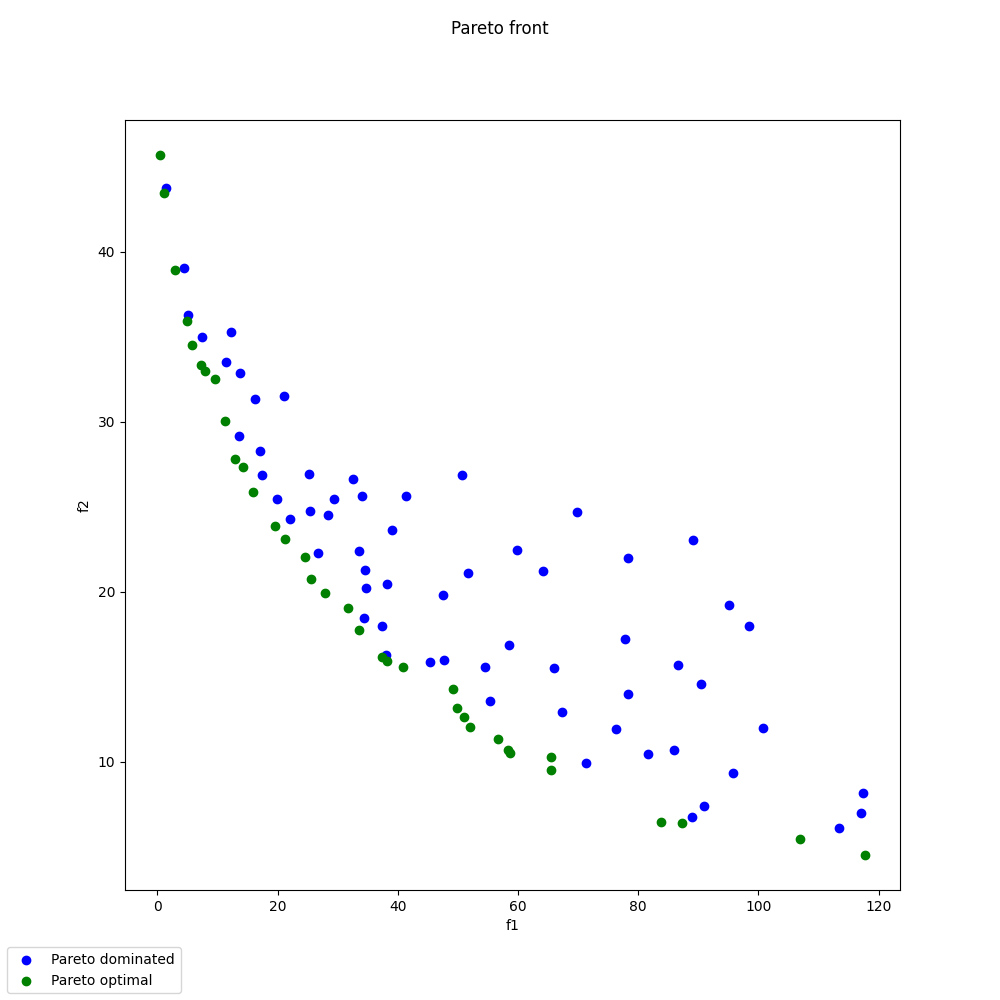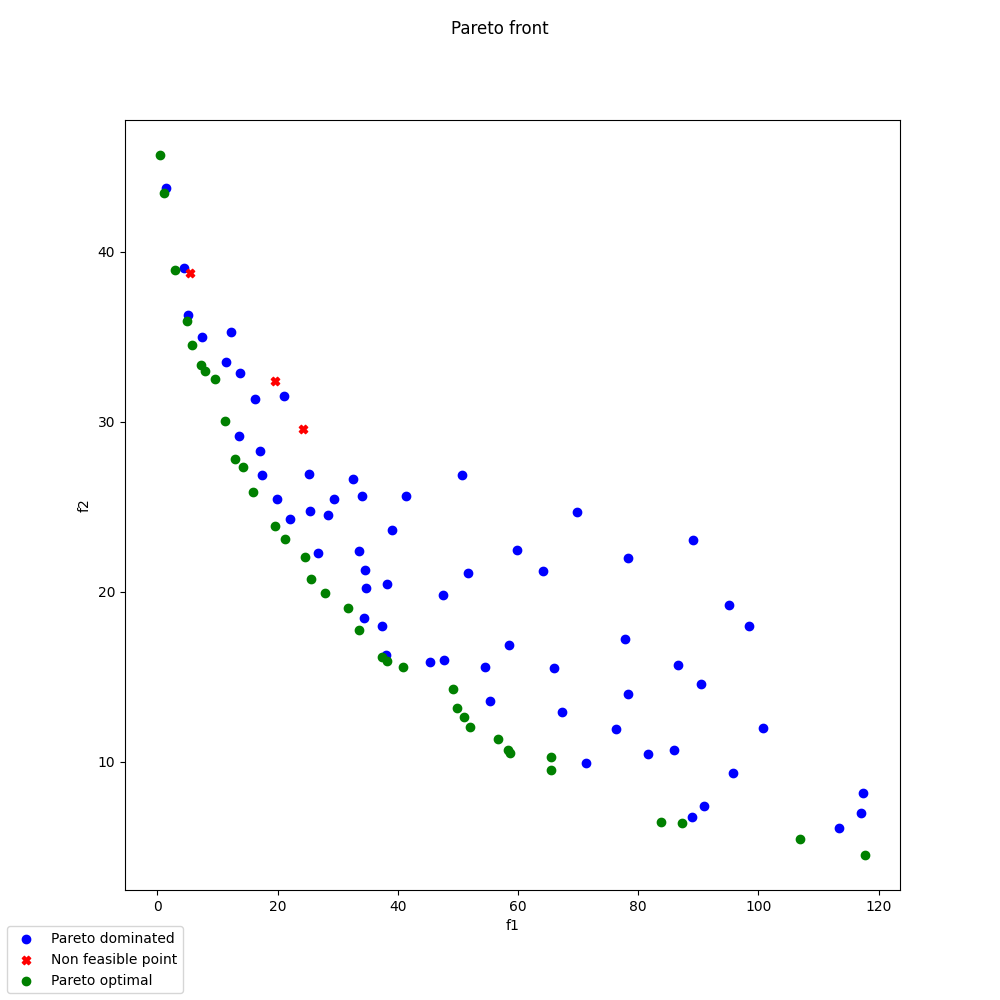# Pareto front on Binh and Korn problem¶

In this example, we illustrate the use of the ParetoFront plot on the Binh and Korn multi-objective problem.

from __future__ import unicode_literals

from matplotlib import pyplot as plt

from gemseo.algos.doe.doe_factory import DOEFactory


## Import¶

The first step is to import some functions from the API and a method to get the design space.

from gemseo.api import configure_logger
from gemseo.post.post_factory import PostFactory
from gemseo.problems.analytical.binh_korn import BinhKorn

configure_logger()


Out:

<RootLogger root (INFO)>


## Import the optimization problem¶

Then, we instantiate the BinkKorn optimization problem.

problem = BinhKorn()


## Create and execute scenario¶

Then, we create a Design of Experiment factory, and we request the execution a a full-factorial DOE using 100 samples

doe_factory = DOEFactory()
doe_factory.execute(problem, algo_name="OT_OPT_LHS", n_samples=100)


Out:

    INFO - 21:51:32: Optimization problem:
INFO - 21:51:32:    Minimize: compute_binhkorn(x, y) = (4*x**2+ 4*y**2, (x-5.)**2 + (y-5.)**2)
INFO - 21:51:32:    With respect to: x, y
INFO - 21:51:32:    Subject to constraints:
INFO - 21:51:32:       ineq1(x, y): (x-5.)**2 + y**2 <= 25. <= 0.0
INFO - 21:51:32:       ineq2(x, y): (x-8.)**2 + (y+3)**2 >= 7.7 <= 0.0
INFO - 21:51:32: Generation of OT_OPT_LHS DOE with OpenTurns
INFO - 21:51:32: DOE sampling:   0%|          | 0/100 [00:00<?, ?it]
INFO - 21:51:32: DOE sampling: 100%|██████████| 100/100 [00:00<00:00, 1826.56 it/sec]
INFO - 21:51:32: Optimization result:
INFO - 21:51:32: Objective value = 30.39964825035985
INFO - 21:51:32: The result is feasible.
INFO - 21:51:32: Status: None
INFO - 21:51:32: Optimizer message: None
INFO - 21:51:32: Number of calls to the objective function by the optimizer: 100
INFO - 21:51:32: Constraints values:
INFO - 21:51:32:    ineq1 = [-11.77907222]
INFO - 21:51:32:    ineq2 = [-38.26307397]
INFO - 21:51:32: Design space:
INFO - 21:51:32: +------+-------------+-------------------+-------------+-------+
INFO - 21:51:32: | name | lower_bound |       value       | upper_bound | type  |
INFO - 21:51:32: +------+-------------+-------------------+-------------+-------+
INFO - 21:51:32: | x    |      0      | 1.542975634014225 |      5      | float |
INFO - 21:51:32: | y    |      0      | 1.269910308585573 |      3      | float |
INFO - 21:51:32: +------+-------------+-------------------+-------------+-------+

Optimization result:
Design variables: [1.54297563 1.26991031]
Objective function: 30.39964825035985
Feasible solution: True


## Post-process scenario¶

Lastly, we post-process the scenario by means of the ParetoFront plot which generates a plot or a matrix of plots if there are more than 2 objectives, plots in blue the locally non dominated points for the current two objectives, plots in green the globally (all objectives) Pareto optimal points. The plots in green denotes non-feasible points. Note that the user can avoid the display of the non-feasible points.

PostFactory().execute(
problem,
"ParetoFront",
save=False,
show=False,
show_non_feasible=False,
objectives=["compute_binhkorn"],
objectives_labels=["f1", "f2"],
)

PostFactory().execute(
problem,
"ParetoFront",
save=False,
show=False,
show_non_feasible=True,
objectives=["compute_binhkorn"],
objectives_labels=["f1", "f2"],
)
# Workaround for HTML rendering, instead of show=True
plt.show()

••Total running time of the script: ( 0 minutes 0.544 seconds)

Gallery generated by Sphinx-Gallery